### Home > MC2 > Chapter 8 > Lesson 8.2.2 > Problem8-43

8-43.

Copy the Diamond Problems below and complete each of them. The pattern used in the Diamond Problems is shown below.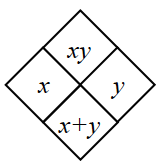1.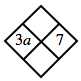$(3a)(7)$
$3a + 7$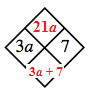1.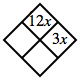$(?)(3x) = 12x$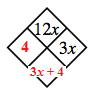1.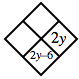$? + 2y = 2y − 6$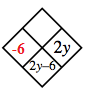1.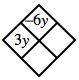$(3y)(?) =-6y$